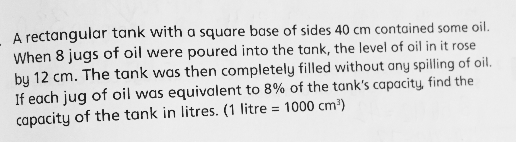# QuestionAnyone help me with attached question

Thanks

Method 1 :
40 x 40 x 12 = 19200 (total capacity of the 8 jugs)
19200/8 = 2400 (capacity of each jug)
8% ——- 2400
1% ——- 2400/8 = 300
100% ——- 100 x 300 = 30000
30000 cubic cm = 30 litres

Method 2 :
12/8 = 1.5 (increase in height of tank after oil in 1 jug was poured into it)
8% ——- 1.5
1% ——- 1.5/8 = 0.1875
100% ——- 100 x 18.75
18.75 x 40 x 40 = 30000
30000 cubic cm = 30 litres

Ans : 30 litres.

0 Replies 1 Like ✔Accepted Answer

We can compute the volume of one jug of oil.

12/8=1.5 cm,  is the height of one jug.

40*40*1.5= 2400 cm^3, is the volume of a single jug of oil.

Then the capacity of the tank is 2400/8%=30000 cm^3=30 L.

0 Replies 1 Like